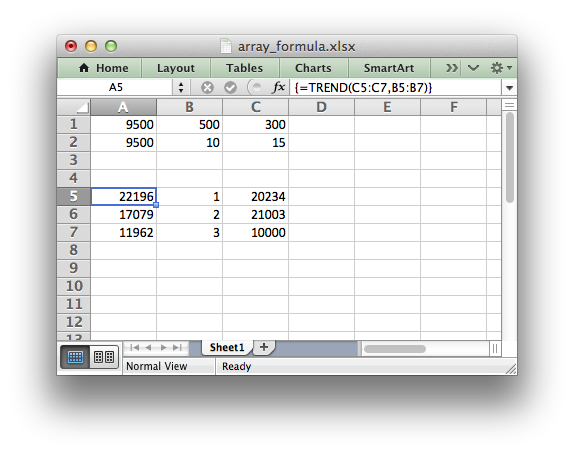# Example: Array formulas

This program is an example of writing array formulas with one or more return values. See the `write_array_formula()` method for more details.```#######################################################################
#
# Example of how to use Python and the XlsxWriter module to write
# simple array formulas.
#
# Copyright 2013-2023, John McNamara, jmcnamara@cpan.org
#
import xlsxwriter

# Create a new workbook and add a worksheet
workbook = xlsxwriter.Workbook("array_formula.xlsx")

# Write some test data.
worksheet.write("B1", 500)
worksheet.write("B2", 10)
worksheet.write("B5", 1)
worksheet.write("B6", 2)
worksheet.write("B7", 3)
worksheet.write("C1", 300)
worksheet.write("C2", 15)
worksheet.write("C5", 20234)
worksheet.write("C6", 21003)
worksheet.write("C7", 10000)

# Write an array formula that returns a single value
worksheet.write_formula("A1", "{=SUM(B1:C1*B2:C2)}")

# Same as above but more verbose.
worksheet.write_array_formula("A2:A2", "{=SUM(B1:C1*B2:C2)}")

# Write an array formula that returns a range of values
worksheet.write_array_formula("A5:A7", "{=TREND(C5:C7,B5:B7)}")

workbook.close()
```